RD Sharma 2013 Solutions for Class 6 Math Chapter 11 Angles are provided here with simple step-by-step explanations. These solutions for Angles are extremely popular among class 6 students for Math Angles Solutions come handy for quickly completing your homework and preparing for exams. All questions and answers from the RD Sharma 2013 Book of class 6 Math Chapter 11 are provided here for you for free. You will also love the ad-free experience on Meritnation’s RD Sharma 2013 Solutions. All RD Sharma 2013 Solutions for class 6 Math are prepared by experts and are 100% accurate.

#### Question 1:

Give two examples each of right, acute and obtuse angles from your environment.

#### Answer:

Two examples of right angle in our environment are:
(i) The angle formed by the two adjacent walls of a room is a right angle.
(ii) The angle formed by the two adjacent edges of a book is a right angle.

Two examples of acute angle in our environment are:
(i) The angle formed between the two adjacent fingers of our hand.
(ii) The angle between the two adjacent sides of the letter Z of English alphabet.

Two examples of obtuse angle in our environment are:
(i) The smaller angle formed by the two adjacent blades of a fan.
(ii) The smaller angle formed by the two sloping sides of a roof of a hut is an obtuse angle.

#### Question 2:

An angle is formed by two adjacent fingers. What kind of angle will it appear?

#### Answer:

Angle formed by two adjacent fingers will appear as an acute angle.

#### Question 3:

Shikha is rowing a boat due north-east. In which direction will she be rowing if she turns it through:

(i) a straight angle
(ii) a complete angle

#### Answer:

(i) If Shikha turns through a straight angle or 180 degrees, she will be rowing along the south-west direction.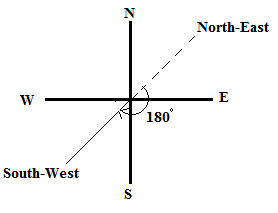(ii) If Shikha turns through a complete angle or 360 degrees, she will be rowing along her original direction, i.e., north-east direction.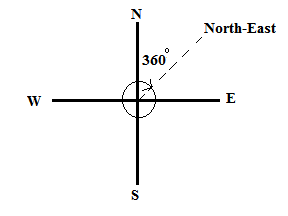#### Question 4:

What is the measure of the angle in degrees between:

(i) North and West?
(ii) North and South?
(iii) North and South-East?

#### Answer:

The measure of the angle between:
(i) North and west is 90o.​
(ii) North and South is 180o.​
(iii) North and South-East is 135o.​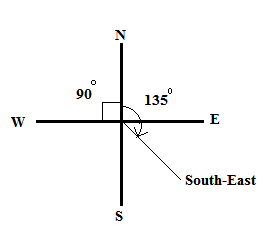#### Question 5:

A ship sailing in river Jhelam moves towards east. If it changes to north, through what angle does it turn?

#### Answer:

If a ship is sailing in east direction and turns to north direction, it turns through an angle of 90 degrees.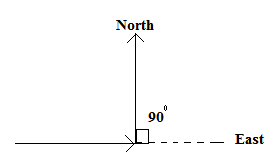#### Question 6:

You are standing in a class-room facing north. In what direction are you facing after making a quarter turn?

#### Answer:

After making a quarter turn or a turn of 90o,​ I will be facing east if I turn to my right hand. Similarly, if I turn to my left hand, I will be facing west.

#### Question 7:

A bicycle wheel makes four and a half turns. Find the number of right angles through which it turns.

#### Answer:

In one turn, the wheel of a bicycle covers 360˚.

If we express 360˚ in right angles, we get:

$\frac{360˚}{90˚}$ = 4 right angles

Thus, in four and a half turns, the wheel will turn by (4 × 4.5) = 18 right angles

#### Question 8:

Look at your watch face. Through how many right angles does the minute-hand moves between 8:00 O' clock and 10:30 O'clock?

#### Answer:

The time interval between 8:00 O'clock and 10:30 O'clock is 2.5 hours, i.e., two and a half hours.

In 1 hour, the minute hand turns by a complete angle, i.e., 360˚ or 360˚/90˚ = 4 right angles.

Thus, in 2.5 hours, the minute hand will turn by 2.5 × 4 = 10 right angles.

#### Question 9:

If a bicycle wheel has 48 spokes, then find the angle between a pair of adjacent spokes.

#### Answer:

In a bicycle, the central angle measures 360˚ and it consists of 48 spokes.

$\therefore$ Angle between any two adjacent spokes = $\frac{360}{48}$ = 7.5˚

#### Question 10:

Classify the following angles as acute, obtuse, straight, right, zero and complete angle:

(i) 118°
(ii) 29°
(iii) 145°
(iv) 165°
(v) 0°
(vi) 75°
(vii) 180°
(viii) 89.5°
(ix) 30°
(x) 90°
(xi) 179°
(xii) 360°
(xiii) $90{\frac{1}{2}}^{0}$

#### Answer:

An acute angle measures between 0° and 90°; an obtuse angle measures between 90° and 180°; a straight angle measures 180°; a right angle measures 90°; a zero angle measures 0° and a complete angle measures 360°.
(i) 118° is an obtuse angle.
(ii) 29° is an acute angle.
(iii) 145° is an obtuse angle.
(iv) 165° is an obtuse angle.
(v) 0° is a zero angle.
(vi) 75° is an acute angle.
(vii) 180° is a straight angle.
(viii) 89.5° is an acute angle.
(ix) 30° is an acute angle.
(x) 90° is a right angle.
(xi) 179° is an obtuse angle.
(xii) 360° is a complete angle.
(xiii) 90$\frac{1}{2}$° is an obtuse angle.

#### Question 11:

Using only a ruler, draw an acute angle, a right angle and an obtuse angle in your notebook and name them.

#### Answer: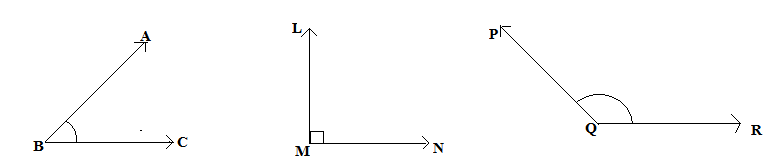Acute angle ∠ABC                        Right angle ∠LMN                 Obtuse angle ∠PQR

#### Question 12:

State the kind of angle, in each case, formed between the following directions:

(i) East and West
(ii) East and North
(iii) North and North-East
(iv) North and South-East

#### Answer:

(i) East and west directions form an angle of 180˚, which is a straight angle.
(ii) East and north directions form an angle of 90˚, which is a right angle.
(iii) North and north-east directions form an angle of 45˚, which is an acute angle.
(iv) North and south-east directions form an angle of 135˚, which is an obtuse angle.

#### Question 13:

State the kind of each of the following angles:#### Answer:

(i) Acute angle, as it measures between 0˚ and 90˚.
(ii) Obtuse angle, as it measures between 90˚ and 180˚.
(iii) Straight angle, as it is equal to 180˚.
(iv) Right angle, as it is equal to 90˚.
(v) Complete angle, as it is equal to 360˚.

#### Question 1:

The vertex of an angles lies

(a) in its interior
(b) in its exterior
(c) on the angle
(d) inside the angle

#### Answer:

(c) on the angle
The vertex of an angle lies on the angle.

#### Question 2:

The figure formed by two rays with the same initial point is known as

(a) a ray
(b) a line
(c) an angle
(d) a line segment

#### Answer:

(c) an angle
An angle is a figure formed by two rays with the same initial point.

#### Question 3:

An angle of measure 0° is called

(a) a complete angle
(b) a right angle
(c) a straight angle
(d) None of these

#### Answer:

(d) None of these
An angle of measure 0° is called a zero angle.

#### Question 4:

An angle of measure 90° is called

(a) a complete angle
(b) a right angle
(c) a straight angle
(d) a reflex angle

#### Answer:

(b) a right angle
An angle of measure 90° is called a right angle.

#### Question 5:

An angle of measure 180° is called

(a) a zero angle
(b) a right angle
(c) a straight angle
(d) a reflex angle

#### Answer:

(c) a straight angle
An angle of measure 180° is called a straight angle.

#### Question 6:

An angle of measure 360° is called

(a) a zero angle
(b) a straight angle
(c) a reflex angle
(d) a complete angle

#### Answer:

(d) a complete angle
An angle of measure 360° is called a complete angle.

#### Question 7:

An angle of measure 240° is

(a) an acute angle
(b) an obtuse angle
(c) a straight angle
(d) a complete angle

#### Answer:

None of the given options are correct.
An angle of measure 240° is called a reflex angle.

#### Question 8:

A reflex angle measures

(a) more than 90° but less than 180°
(b) more than 180° but less than 270°
(c) more than 180° but less than 360°
(d) None of these

#### Answer:

(c) more than 180° but less than 360°
A reflex angle is defined as an angle that measures more than 180° but less than 360°.

#### Question 9:

The number of degrees in 2 right angle is

(a) 90°
(b) 180°
(c) 270°
(d) 360°

#### Answer:

(b) 180°

$\because$ 1 right angle = 90°

$\therefore$ 2 right angles = 90°​ × 2 = 180°

#### Question 10:

The number of degrees in $\frac{3}{2}$ right angles is
(a) 180°
(b) 360°
(c) 270°
(d) 90°

#### Answer:

None of the options are correct.

The correct answer is 135°.

$\because$ 1 right angle = 90°

$\therefore$ $\frac{3}{2}$ right angles =  $\frac{3}{2}$ × 90° = 135°

#### Question 11:

If a bicycle wheel has 36 spokes, then the angle between a pair of adjacent spokes is

(a) 10°
(b) 15°
(c) 20°
(d) 12°

#### Answer:

(a) 10°

The complete central angle of a bicycle wheel measures 360°.

Therefore, the angle between two adjacent spokes of the wheel containing 36 spokes = $\frac{360}{36}$ = 10°

#### Question 1:

Give three examples of angles from your environment.

#### Answer:

Three examples of angles from our environment are:
(i) Angle formed by the minute and hour hands of an analog clock
(ii) Angle formed by the two adjacent walls of a room
(iii) Angle formed by the two adjacent fingers of our hand

#### Question 2:

Write the arms and the vertex of ∠LMP given in Fig. 11.14.#### Answer:

Arms of ∠LMP are MP and ML. Further, its vertex is M.

#### Question 3:

How many angles are formed in the figures 11.15 (i), (ii) and (iii)? Name them.#### Answer:

(i) Three angles are formed, namely ∠ABC, ∠BAC and ∠ACB.
(ii) Four angles are formed, namely ∠ABC, ∠ADC, ∠BCD and ∠BAD.
(iii) Eight angles are formed namely, ∠ADC, ∠ACD, ∠DAC, ∠ACB, ∠ABC, ∠BAC, ∠BCD and ∠BAD.

#### Question 4:

In Fig. 11.16, list the points which are: (i) in the interior of ∠P (ii) in the exterior of ∠P and (iii) lie on ∠P.#### Answer:

(i) Points J and C lie in the interior of ∠P.
(ii) Points D and B lie in the exterior of ∠P.
(iii) Points A, P and M lie on ∠P.

#### Question 5:

In Fig 11.17, write another name for:(i) ∠1
(ii) ∠2
(iii) ∠3
(iv) ∠4

#### Answer:

(i) Another name for ∠1 is ∠BOD.
(ii) Another name for ∠2 is ∠BOC.
(iii) Another name for ∠3 is ∠AOC.
(iv) Another name for ∠4 is ∠ AOD.

#### Question 6:

In fig. 11.18, Write another name for:(i) ∠1
(ii) ∠2
(iii) ∠3

(i) ∠BPE
(ii) ∠PQC
(iii) ∠DQF

#### Question 7:

In Fig. 11.19, Which of the following statement are true:(i) Point B is the interior of ∠AOB
(ii) Point B is the interior of ∠AOC
(iii) Point A is the interior of ∠AOD
(iv) Point C is the exterior of ∠AOB
(v) Point D si the exterior of ∠AOC

#### Answer:

(ii), (iv) and (v) are true statements.
(i) and (iii) are incorrect statements as B lies on ∠AOB and A lies on ∠AOD.

#### Question 8:

Which of the following statements are true:

(i) The vertex of an angle lies in its interior.
(ii) The vertex of an angle lies in its exterior.
(iii) The vertex of an angle lies on it.

#### Answer:

(iii) The vertex of an angle lies on it.
This is the only correct statement.

#### Question 9:

By simply looking at the pair of angles given in Fig. 11.20, state which of the angles in each of the pairs is greater:#### Answer:

(i) ∠AOB is greater than ∠DEF.
(ii) ∠PQR is greater than ∠LMN.
(iii) ∠UVW is greater than ∠XYZ.

#### Question 10:

By using tracing paper compare the angles in each of the pairs given in Fig. 11.21.#### Answer:

Using tracing paper, we get that:
(i)∠PQR is greater than ∠AOB.
(ii) ∠UVW is greater than ∠LMN.
(iii) ∠RST is greater than ∠XYZ.
(iv) ∠PQR is greater than ∠EFG.

View NCERT Solutions for all chapters of Class 6